# Mensuration Quiz for IBPS , SBI , RBI: Quiz – 1

## Mensuration Quiz for IBPS , SBI , RBI: Quiz – 1

Mensuration plays a significant role in Quantitative Aptitude Section of banking exams such as IBPS, SBI and RBI PO and Clerk. You will get at least 2-3 questions from Mensuration in one of IBPS, SBI and RBI PO & clerk exam. So, aspirants should focus on Mensuration questions in detail. Here, we are providing you with the Mensuration questions quiz with the detailed solution so that you can easily prepare for Mensuration questions. We are providing here all-important latest pattern-based questions and Previous Year Questions of Mensuration of various Government Exam like IBPS, SBI, and RBI PO and Clerk exam. This Mensuration quiz we are providing is free. Attempt this Mensuration quiz to practice important questions with answers and solutions. And score better in IBPS, SBI and RBI PO and Clerk exam.

Mensuration Quiz to improve your Quantitative Aptitude for SBI Po & SBI clerk exam, IBPS PO & IBPS Clerk exam , IBPS RRB PO and assistant exam, LIC AAO ,LIC Assistant  and other competitive exam.

1. Diameter of a circle is 28 cm and sum of circumference of that circle and area of a square is 257 sq. cm. Find the perimeter of square?

(a) 56cm

(b) 52cm

(c) 64cm

(d) 58cm

(e) 48cm2. Circumference of a circular garden is 88 cm and area of circular garden is 132 sq.cm more than area of a square hall. Find the perimeter of the square hall?

(a) 68cm

(b) 78cm

(c) 88cm

(d) 76cm

(e) 72cm3. Radius of two circles are in the ratio of 1 : 3. Sum of circumference of both circles is 176 cm, which is equal to perimeter of a rectangle. If ratio between length to breadth of rectangle is 8 : 3, then what is the difference between radius of bigger circle and length of rectangle?

(a) 60 cm

(b) 65 cm

(c) 62 cm

(d) 43 cm

(e) 59 cm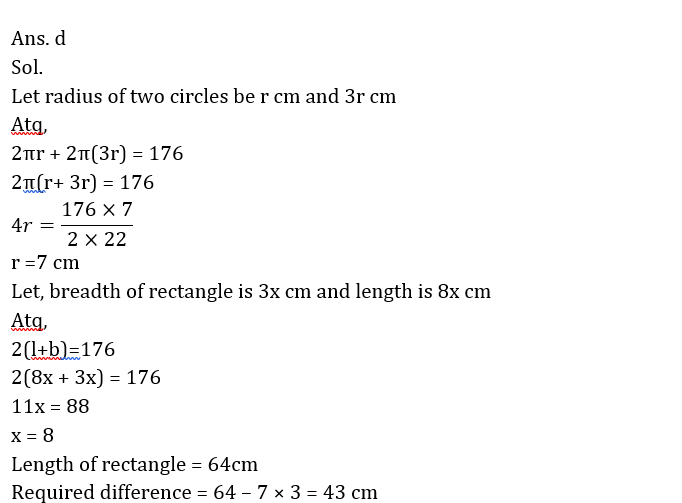4. A spherical cannon ball of diameter 24 cm is melted and casted into two cylinders of equal size and shape having base radius 8 cm. Find the height of each cylinder?

(a) 36 cm

(b) 18 cm

(c) 32 cm

(d) 20 cm

(e) 16 cm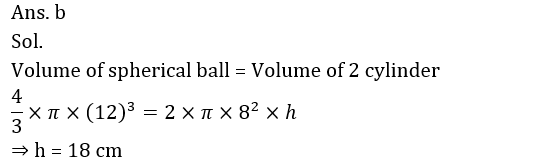5. A path of width 1.5 m is to be built around a rectangular park of length 12 m and breadth 9 m. Find the area of the rectangular path

(a) 72 m2

(b) 60 m2

(c) 75 m2

(d) 80 m2

(e) 65 m2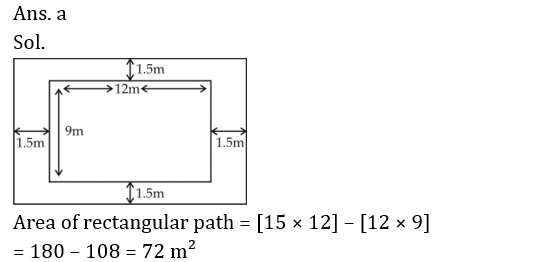6. Find the ratio of volume of cylinder ‘A’ to volume of cylinder ‘B’, if ratio of radius of cylinders ‘A’ to cylinder ‘B’ is 1 : 2 while the ratio of height of cylinder ‘A’ to cylinder ‘B’ is 2 : 1.

(a) 1 : 1

(b) 1 : 2

(c) 2 : 1

(d) 1 : 4

(e) 1 : 8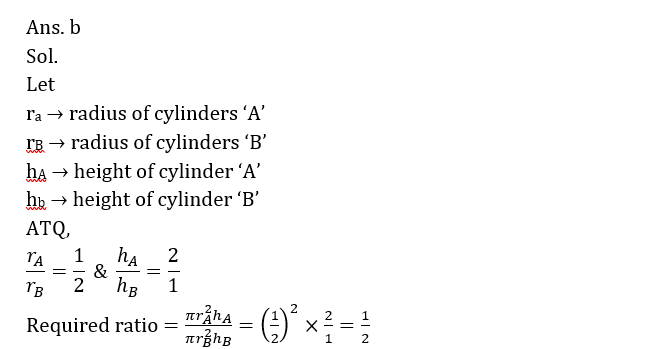1. Find the height of equilateral triangle whose area issq. cm. ?8. A sphere of radius 8 cm is melted to form 8 cylinder of radius 4 cm. find height of cylinder?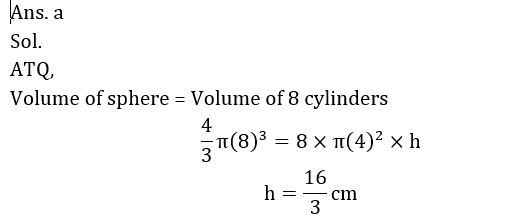9. Sum of area of circle and rectangle is 1264 sq. cm. The diameter of the circle is 28 cm then what will be the sum of circumference of the circle and the perimeter of the rectangle, if length of rectangle is two times of breath?

(a) 144 cm

(b) 196 cm

(c) 136 cm

(d) 164 cm

(e) 256 cm10. Ratio of radius to height of a cylinder is 1 : 8. If the height of cylinder is reduced by 25/2% then find p.#### Attempt Quantitative Aptitude Topic Wise Online Test Series

Recommended PDF’s for 2021:

### 2021 Preparation Kit PDF

#### Most important PDF’s for Bank, SSC, Railway and Other Government Exam : Download PDF Now

AATMA-NIRBHAR Series- Static GK/Awareness Practice Ebook PDF Get PDF here
The Banking Awareness 500 MCQs E-book| Bilingual (Hindi + English) Get PDF here
AATMA-NIRBHAR Series- Banking Awareness Practice Ebook PDF Get PDF here
Computer Awareness Capsule 2.O Get PDF here
AATMA-NIRBHAR Series Quantitative Aptitude Topic-Wise PDF 2020 Get PDF here
Memory Based Puzzle E-book | 2016-19 Exams Covered Get PDF here
Caselet Data Interpretation 200 Questions Get PDF here
Puzzle & Seating Arrangement E-Book for BANK PO MAINS (Vol-1) Get PDF here
ARITHMETIC DATA INTERPRETATION 2.O E-book Get PDF here

3